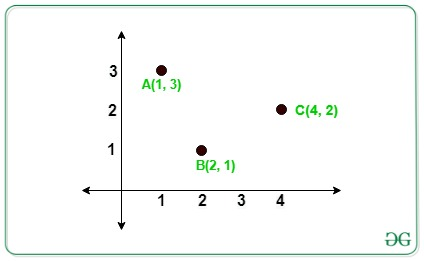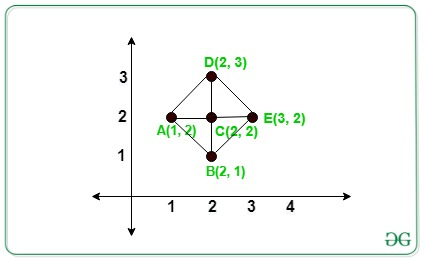# Count of Right-Angled Triangle formed from given N points whose base or perpendicular are parallel to X or Y axis

• Difficulty Level : Hard
• Last Updated : 11 Jul, 2022

Given an array arr[] of N distinct integers points on the 2D Plane. The task is to count the number of Right-Angled Triangle from N points such that the base or perpendicular is parallel to the X or Y-axis.

Examples:

Input: arr[][] = {{4, 2}, {2, 1}, {1, 3}}
Output:
Explanation:In the above image there is no right-angled triangle formed.
Input: arr[][] = {{1, 2}, {2, 1}, {2, 2}, {2, 3}, {3, 2}}
Output:
Explanation:In the above image there are 4 right-angled triangles formed by triangles ACB, ACD, DCE, BCE.

Approach: The idea is to store the count of each co-ordinate’s having the same X and Y co-ordinates respectively. Now traverse each given points and the count of a right-angled triangle formed by each coordinate (X, Y) is given by:

Count of right-angled triangles = (frequencies of X coordinates – 1) * (frequencies of Y coordinates – 1)

Below are the steps:

• Create two maps to store the count of points, one for having the same X-coordinate and another for having the same Y-coordinate.
• For each value in the map of x-coordinate and in the map of y-coordinate choose that pair of points as pivot elements and find the frequency of that pivot element.
• For each pivot element(say pivot) in the above step, the count of right-angled is given by:

(m1[pivot].second-1)*(m2[pivot].second-1)

• Similarly, calculate the total possible right-angled triangle for other N points given.
• Finally, sum all the possible triangle obtained that is the final answer.

Below is the implementation of the above approach:

## C++

 `// C++ program for the above approach`   `#include ` `using` `namespace` `std;`   `// Function to find the number of right` `// angled triangle that are formed from` `// given N points whose perpendicular or` `// base is parallel to X or Y axis` `int` `RightAngled(``int` `a[], ``int` `n)` `{`   `    ``// To store the number of points` `    ``// has same x or y coordinates` `    ``unordered_map<``int``, ``int``> xpoints;` `    ``unordered_map<``int``, ``int``> ypoints;`   `    ``for` `(``int` `i = 0; i < n; i++) {` `        ``xpoints[a[i]]++;` `        ``ypoints[a[i]]++;` `    ``}`   `    ``// Store the total count of triangle` `    ``int` `count = 0;`   `    ``// Iterate to check for total number` `    ``// of possible triangle` `    ``for` `(``int` `i = 0; i < n; i++) {`   `        ``if` `(xpoints[a[i]] >= 1` `            ``&& ypoints[a[i]] >= 1) {`   `            ``// Add the count of triangles` `            ``// formed` `            ``count += (xpoints[a[i]] - 1)` `                     ``* (ypoints[a[i]] - 1);` `        ``}` `    ``}`   `    ``// Total possible triangle` `    ``return` `count;` `}`   `// Driver Code` `int` `main()` `{` `    ``int` `N = 5;`   `    ``// Given N points` `    ``int` `arr[] = { { 1, 2 }, { 2, 1 },` `                     ``{ 2, 2 }, { 2, 3 },` `                     ``{ 3, 2 } };`   `    ``// Function Call` `    ``cout << RightAngled(arr, N);`   `    ``return` `0;` `}`

## Python3

 `# Python3 program for the above approach ` `from` `collections ``import` `defaultdict`   `# Function to find the number of right ` `# angled triangle that are formed from ` `# given N points whose perpendicular or ` `# base is parallel to X or Y axis ` `def` `RightAngled(a, n):` `    `  `    ``# To store the number of points ` `    ``# has same x or y coordinates ` `    ``xpoints ``=` `defaultdict(``lambda``:``0``)` `    ``ypoints ``=` `defaultdict(``lambda``:``0``)` `    `  `    ``for` `i ``in` `range``(n):` `        ``xpoints[a[i][``0``]] ``+``=` `1` `        ``ypoints[a[i][``1``]] ``+``=` `1` `        `  `    ``# Store the total count of triangle ` `    ``count ``=` `0` `    `  `    ``# Iterate to check for total number ` `    ``# of possible triangle ` `    ``for` `i ``in` `range``(n):` `        ``if` `(xpoints[a[i][``0``]] >``=` `1` `and` `            ``ypoints[a[i][``1``]] >``=` `1``):` `            `  `            ``# Add the count of triangles ` `            ``# formed ` `            ``count ``+``=` `((xpoints[a[i][``0``]] ``-` `1``) ``*` `                      ``(ypoints[a[i][``1``]] ``-` `1``))` `            `  `    ``# Total possible triangle` `    ``return` `count`   `# Driver Code ` `N ``=` `5`   `# Given N points ` `arr ``=` `[ [ ``1``, ``2` `], [ ``2``, ``1` `],` `        ``[ ``2``, ``2` `], [ ``2``, ``3` `],` `        ``[ ``3``, ``2` `] ]`   `# Function call` `print``(RightAngled(arr, N))`   `# This code is contributed by Stuti Pathak`

## Java

 `// Java program for the above approach` `import` `java.util.*;` `class` `GFG{`   `// Function to find the number of right` `// angled triangle that are formed from` `// given N points whose perpendicular or` `// base is parallel to X or Y axis` `static` `int` `RightAngled(``int` `a[][], ``int` `n)` `{`   `    ``// To store the number of points` `    ``// has same x or y coordinates` `    ``HashMap xpoints  = ``new` `HashMap();` `    ``HashMap ypoints  = ``new` `HashMap();`   `    ``for` `(``int` `i = ``0``; i < n; i++) ` `    ``{` `        ``if``(xpoints.containsKey(a[i][``0``]))` `        ``{` `            ``xpoints.put(a[i][``0``], xpoints.get(a[i][``0``]) + ``1``);` `        ``}` `        ``else` `        ``{` `            ``xpoints.put(a[i][``0``], ``1``);` `        ``}` `        ``if``(ypoints.containsKey(a[i][``1``]))` `        ``{` `            ``ypoints.put(a[i][``1``], ypoints.get(a[i][``1``]) + ``1``);` `        ``}` `        ``else` `        ``{` `            ``ypoints.put(a[i][``1``], ``1``);` `        ``}` `    ``}`   `    ``// Store the total count of triangle` `    ``int` `count = ``0``;`   `    ``// Iterate to check for total number` `    ``// of possible triangle` `    ``for` `(``int` `i = ``0``; i < n; i++)` `    ``{` `        ``if` `(xpoints.get(a[i][``0``]) >= ``1` `&&` `            ``ypoints.get(a[i][``1``]) >= ``1``)` `        ``{`   `            ``// Add the count of triangles` `            ``// formed` `            ``count += (xpoints.get(a[i][``0``]) - ``1``) *` `                     ``(ypoints.get(a[i][``1``]) - ``1``);` `        ``}` `    ``}`   `    ``// Total possible triangle` `    ``return` `count;` `}`   `// Driver Code` `public` `static` `void` `main(String[] args)` `{` `    ``int` `N = ``5``;`   `    ``// Given N points` `    ``int` `arr[][] = { { ``1``, ``2` `}, { ``2``, ``1` `},` `                    ``{ ``2``, ``2` `}, { ``2``, ``3` `},` `                    ``{ ``3``, ``2` `} };`   `    ``// Function Call` `    ``System.out.print(RightAngled(arr, N));` `}` `}`   `// This code is contributed by Rajput-Ji`

## C#

 `// C# program for the above approach` `using` `System;` `using` `System.Collections.Generic;` `class` `GFG{`   `    ``// Function to find the number of right` `    ``// angled triangle that are formed from` `    ``// given N points whose perpendicular or` `    ``// base is parallel to X or Y axis` `    ``static` `int` `RightAngled(``int``[, ] a, ``int` `n)` `    ``{`   `        ``// To store the number of points` `        ``// has same x or y coordinates` `        ``Dictionary<``int``, ``int``> xpoints = ``new` `Dictionary<``int``, ``int``>();` `        ``Dictionary<``int``, ``int``> ypoints = ``new` `Dictionary<``int``, ``int``>();`   `        ``for` `(``int` `i = 0; i < n; i++) ` `        ``{` `            ``if` `(xpoints.ContainsKey(a[i, 0])) ` `            ``{` `                ``xpoints[a[i, 0]] = xpoints[a[i, 0]] + 1;` `            ``}` `            ``else` `            ``{` `                ``xpoints.Add(a[i, 0], 1);` `            ``}` `            ``if` `(ypoints.ContainsKey(a[i, 1])) ` `            ``{` `                ``ypoints[a[i, 1]] = ypoints[a[i, 1]] + 1;` `            ``}` `            ``else` `            ``{` `                ``ypoints.Add(a[i, 1], 1);` `            ``}` `        ``}`   `        ``// Store the total count of triangle` `        ``int` `count = 0;`   `        ``// Iterate to check for total number` `        ``// of possible triangle` `        ``for` `(``int` `i = 0; i < n; i++) ` `        ``{` `            ``if` `(xpoints[a[i, 0]] >= 1 && ` `                ``ypoints[a[i, 1]] >= 1) ` `            ``{`   `                ``// Add the count of triangles` `                ``// formed` `                ``count += (xpoints[a[i, 0]] - 1) * ` `                         ``(ypoints[a[i, 1]] - 1);` `            ``}` `        ``}`   `        ``// Total possible triangle` `        ``return` `count;` `    ``}`   `    ``// Driver Code` `    ``public` `static` `void` `Main(String[] args)` `    ``{` `        ``int` `N = 5;`   `        ``// Given N points` `        ``int``[, ] arr = {{1, 2}, {2, 1}, ` `                       ``{2, 2}, {2, 3}, {3, 2}};`   `        ``// Function Call` `        ``Console.Write(RightAngled(arr, N));` `    ``}` `}`   `// This code is contributed by Rajput-Ji`

## Javascript

 ``

Output:

`4`

Time Complexity: O(N+N) i.e O(N)
Auxiliary Space: O(N+N) i.e O(N)

My Personal Notes arrow_drop_up
Recommended Articles
Page :